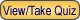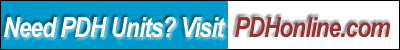Print this page

Electrical Fundamentals –Reactance and Impedance

A. Bhatia, B.E.

Course Outline

“Resistance” is to DC circuits and “Resistance and Reactance” is to AC circuits.

The fact that the voltage of AC current varies sinusoidal with time requires the introduction or modification of the quantities required to take this fact into account. Reactance (X) is such a quantity. It is a measure of the resistance offered by a material or a circuit to the flow of current, but only when the current is alternating.

This 4-hr course material provides insight to the basic concepts of reactance and impedance and is based entirely on Naval Education and Training Materials (NAVEDTRA 14173), Electricity and Electronic Training Series; Module-2, Chapter 4 titled “Inductive and Capacitive Reactance”.

The course includes a multiple-choice quiz at the end, which is designed to enhance the understanding of course materials.

Learning Objective

At the conclusion of this course, the student will be able to:

• State the effects an inductor has on a change in current and a capacitor has on a change in voltage;
• State the phase relationships between current and voltage in an inductor and in a capacitor;
• Understand the formulas for calculating inductive and capacitive reactances;
• State the effects of a change in frequency on XL and XC;
• Understand the formula for determining total reactance (X); compute total reactance (X) in a series circuit and indicate whether the total reactance is capacitive or inductive;
• Understand the formula for impedance, and calculate the impedance in a series circuit when the values of XC, X L, and R are given;
• Define true power, reactive power, and apparent power; state the unit of measurement for and the formula used to calculate each;
• State the definition of and write the formula for power factor; and
• State the difference between calculating impedance in a series ac circuit and in a parallel ac circuit.

Intended Audience

This course is aimed at students, professional electrical & electronics engineers, service technicians, energy auditors, operational & maintenance personnel, facility engineers and general audience.

Course Introduction

Reactance is the property of resisting or impeding the flow of AC current or AC voltage in inductors and capacitors. There are two types of reactance, inductive (XL) and capacitive (XC). The first is associated with the magnetic field induced around a coil or wire through which AC current flows, also called an inductance. The other is the capacitive reactance is associated with the changing electric field between two parallel conducting plates separated by an insulator. Two elements of reactance: inductance and capacitance correspond to frequency. Inductance is in proportion to frequency while capacitance is inversely proportional to frequency.

Once you have determined the inductance and the capacitance, you can determine overall how efficiently the current is making it across the circuit, or the reactance. When you combine, or add, the reactance to resistance, you arrive at impedance. Mathematically, Z=V/I, where Z is impedance, given in ohms; V is voltage, given in volts; and I is current, given in amps.

Course Content

In this course, you are required to study Naval Education and Training Materials (NAVEDTRA 14173), Electricity and Electronic Training Series; Module-2, Chapter 4 titled “Inductive and Capacitive Reactance”:

Inductive and Capacitive Reactance (Chapter 4, NAVEDTRA 14173)

Please click on the above underlined hypertexts to view, download or print the documents for your study. Because of the large file size, we recommend that you first save the file to your computer by right clicking the mouse and choosing "Save Target As ...", and then open the file in Adobe Acrobat Reader.

Course Summary

Alternating current (AC) circuits involve potential differences and currents which vary sinusoidal with time. Time dependent electromagnetic effects are persistent in AC circuits and are described by term Reactance (X). Reactance is the property of resisting or impeding the flow of AC current or AC voltage in inductors and capacitors.

The inductive reactance is a measure of the resistance to the flow of this current and it is proportional to the frequency of the alternating current and to inductance (L), an inductor property arising from Faraday's law, and defined in terms of the electromotive force (emf) generated to oppose a given change in current. Inductive reactance is determined by the formula:

2 * pi * f * L

Where

2 * pi = 6.2832, f = frequency in hertz and L = inductance in Henries

The capacitive reactance is a measure of this resistance and is inversely proportional to the frequency of the alternating current and to capacitance (C), a capacitor property equal to the magnitude of the charge stored on each capacitor plate (Q) divided by the voltage applied to the plates. Capacitive reactance is determined by the formula:

1 / (2 * pi * f * C)

Where:

2 * pi = 6.2832; f = frequency in hertz and C = capacitance in Farads

Impedance consists of the sum of resistance and reactance contributions. The resistance contribution results from the collisions of the electron-carrying current-with the constituent atoms of the conductor material. The reactance component is an additional resistance to the movement of electric charge resulting from the changing magnetic and electric fields occurring in AC circuits. From the modified Ohm's law, the impedance of a circuit is equal to the voltage measured across the circuit, divided by the maximum value of the current through the circuit (Z = V/I). Impedance, like resistance, is expressed in ohms.
Impedance has two parts. The resistive or real part represents power consumption. The reactive or imaginary part represents power storage. Ohm's Law can be used for AC analysis, providing that impedance is used in place of resistance:

V = IZ        I = V/Z        Z = V/I

In a resistor, voltage and current are in phase, so effectively, Z= R. In a capacitor, voltage lags current by 90° and in an inductor; voltage leads current by 90°. Thus, when an AC circuit includes capacitors or inductors, even though Z is also the ratio of the voltage and current peaks, as is the case for R in the DC formulation of Ohm's law, the I and V peaks are now out-of-phase, that is, they do not occur at the same time. The impedance (Z) is a phase angle, which describes the angle between the current and voltage AC sinusoidal curves.

Series RLC Circuit- Current is the same in any series circuit. This major characteristic must always be remembered as you solve for series circuit values. Opposition to this current is impedance. In a series RLC circuit, impedance consists of resistance and reactance.

Parallel RLC Circuit - When resistors, inductors, and capacitors are connected in parallel, voltage remains as the reference, is equal, and is in phase across all components. However, it should be noted that it is current that changes. With each component it is in phase, or leads, or lags.

Quiz

Once you finish studying the above course content, you need to take a quiz to obtain the PDH credits.DISCLAIMER: The materials contained in the online course are not intended as a representation or warranty on the part of PDH Center or any other person/organization named herein. The materials are for general information only. They are not a substitute for competent professional advice. Application of this information to a specific project should be reviewed by a registered architect and/or professional engineer/surveyor. Anyone making use of the information set forth herein does so at their own risk and assumes any and all resulting liability arising therefrom.